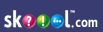Single Entry and Incomplete Records Generix Content - Single Entry and Incomplete Records
"Single Entry and Incomplete Records" question number distribution across years
loading...
Financial Accounting index on skoool nigeriaWAEC questions for "Financial Accounting :: Single Entry and Incomplete Records"
Q1

An approach for discovering missing figures in incomplete records is the use of

A

cash book

B

control accounts

C

trading accounts

D

balance sheet

E
Q2

In single entry and incomplete records, the net assets method used to ascertain profits is

A

net asset at the end of a period - net assets at the beginning of a profit = profit + additional capital - drawing

B

net asset at the end of a period let asset at the beginning of a period = profit - additional capital -drawings.

C

net assets at the end of a period - net assets at the beginning of a period - drawings.

D

net asset at the end of a period + additional capital - drawings

E
Q3

Which of the problem is not used in solving problems of incomplete records?

A

control accounts

B

statement of affairs

C

cash book

D

balance sheet

E
Q4

In a system of incomplete records the opening capital is determined by preparing

A

Statement of affairs

B

Balance sheet

C

Cash book

D

Trial balance

E
Q5

Which of the following is used to derive missing figures in a system of incomplete records?

A

control account

B

bank account

C

profit and loss account

D

bank statement

E